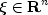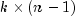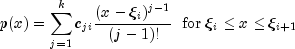JMSLTM Numerical Library 7.2.0
com.imsl.math

## Class Spline

• All Implemented Interfaces:
Serializable, Cloneable
Direct Known Subclasses:
CsAkima, CsInterpolate, CsPeriodic, CsShape, CsSmooth, CsSmoothC2, CsTCB

```public abstract class Spline
extends Object
implements Serializable, Cloneable```
Spline represents and evaluates univariate piecewise polynomial splines.

A univariate piecewise polynomial (function) p(x) is specified by giving its breakpoint sequence, the order k (degree k-1) of its polynomial pieces, and thematrix c of its local polynomial coefficients. In terms of this information, the piecewise polynomial (ppoly) function is given byThe breakpoint sequenceis assumed to be strictly increasing, and we extend the ppoly function to the entire real axis by extrapolation from the first and last intervals.
Akima Example, Interpolate Example, Periodic Example, Shape Preserving Example, Smoothing Example 1, Smoothing Example 2, Serialized Form
• ### Field Summary

Fields
Modifier and Type Field and Description
`protected double[]` `breakPoint`
The breakpoint array of length n, where n is the number of piecewise polynomials.
`protected double[][]` `coef`
Coefficients of the piecewise polynomials.
`protected static double` `EPSILON_LARGE`
The largest relative spacing for double.
• ### Constructor Summary

Constructors
Constructor and Description
`Spline()`
• ### Method Summary

Methods
Modifier and Type Method and Description
`protected void` ```copyAndSortData(double[] xData, double[] yData)```
Copy and sort xData into breakPoint and yData into the first column of coef.
`protected void` ```copyAndSortData(double[] xData, double[] yData, double[] weight)```
Copy and sort xData into breakPoint and yData into the first column of coef.
`double` `derivative(double x)`
Returns the value of the first derivative of the spline at a point.
`double[]` ```derivative(double[] x, int ideriv)```
Returns the value of the derivative of the spline at each point of an array.
`double` ```derivative(double x, int ideriv)```
Returns the value of the derivative of the spline at a point.
`double[]` `getBreakpoints()`
Returns a copy of the breakpoints.
`double` ```integral(double a, double b)```
Returns the value of an integral of the spline.
`double` `value(double x)`
Returns the value of the spline at a point.
`double[]` `value(double[] x)`
Returns the value of the spline at each point of an array.
• ### Methods inherited from class java.lang.Object

`clone, equals, finalize, getClass, hashCode, notify, notifyAll, toString, wait, wait, wait`
• ### Field Detail

• #### breakPoint

`protected double[] breakPoint`
The breakpoint array of length n, where n is the number of piecewise polynomials.
• #### coef

`protected double[][] coef`
Coefficients of the piecewise polynomials. This is an n by k array, where n is the number of piecewise polynomials and k is the order (degree+1) of the piecewise polynomials.

`coef[i]` contains the coefficients for the piecewise polynomial valid in the interval [`x[k]`,`x[k+1]`).

• #### EPSILON_LARGE

`protected static final double EPSILON_LARGE`
The largest relative spacing for double.
Constant Field Values
• ### Constructor Detail

• #### Spline

`public Spline()`
• ### Method Detail

• #### copyAndSortData

```protected void copyAndSortData(double[] xData,
double[] yData)```
Copy and sort xData into breakPoint and yData into the first column of coef.
• #### copyAndSortData

```protected void copyAndSortData(double[] xData,
double[] yData,
double[] weight)```
Copy and sort xData into breakPoint and yData into the first column of coef.
• #### derivative

`public double derivative(double x)`
Returns the value of the first derivative of the spline at a point.
Parameters:
`x` - a `double`, the point at which the derivative is to be evaluated
Returns:
a `double` containing the value of the first derivative of the spline at the point x
• #### derivative

```public double[] derivative(double[] x,
int ideriv)```
Returns the value of the derivative of the spline at each point of an array.
Parameters:
`x` - a `double` array of points at which the derivative is to be evaluated
`ideriv` - an `int` specifying the derivative to be computed. If zero, the function value is returned. If one, the first derivative is returned, etc.
Returns:
a `double` array containing the value of the derivative of the spline at each point of the array x
• #### derivative

```public double derivative(double x,
int ideriv)```
Returns the value of the derivative of the spline at a point.
Parameters:
`x` - a `double`, the point at which the derivative is to be evaluated
`ideriv` - an `int` specifying the derivative to be computed. If zero, the function value is returned. If one, the first derivative is returned, etc.
Returns:
a `double` containing the value of the derivative of the spline at the point x
• #### getBreakpoints

`public double[] getBreakpoints()`
Returns a copy of the breakpoints.
Returns:
a `double` array containing a copy of the breakpoints
• #### integral

```public double integral(double a,
double b)```
Returns the value of an integral of the spline.
Parameters:
`a` - a `double` specifying the lower limit of integration
`b` - a `double` specifying the upper limit of integration
Returns:
a `double`, the integral of the spline from a to b
• #### value

`public double value(double x)`
Returns the value of the spline at a point.
Parameters:
`x` - a `double`, the point at which the spline is to be evaluated
Returns:
a `double` giving the value of the spline at the point x
• #### value

`public double[] value(double[] x)`
Returns the value of the spline at each point of an array.
Parameters:
`x` - a `double` array of points at which the spline is to be evaluated
Returns:
a `double` array containing the value of the spline at each point of the array x
JMSLTM Numerical Library 7.2.0